# 栈的实现

## 通过数组实现

Java 中，栈（Stack 类）就是通过数组来实现的，下面我们就自己利用数组来实现一个简单的栈：

package com.lonely.wolf.note.stack;

import java.util.Arrays;

/**
* 基于数组来实现自定义栈
*/
public class MyStackByArray<E> {
public static void main(String[] args) {
MyStackByArray stack = new MyStackByArray();
stack.push(1);
System.out.println("stack有效元素个数：" + stack.size);//输出 1
System.out.println("查看栈顶元素：" + stack.peek());//输出 1
System.out.println("栈是否为空：" + stack.isEmpty());//输出 false
System.out.println("弹出栈顶元素：" + stack.pop());// 输出 1
System.out.println("栈是否为空：" + stack.isEmpty());//输出 true
stack.push(2);
stack.push(3);
stack.push(4);
System.out.println("stack有效元素个数：" + stack.size);//输出 3
System.out.println("弹出栈顶元素：" + stack.pop()); //输出 4
}

private Object[] element;//存储元素的数组
private int size;//栈内有效元素
private int DEFAULT_SIZE = 2;//默认数组大小

public MyStackByArray() {
element = new Object[DEFAULT_SIZE];
}

/**
* 判断是否为空，注意不能直接用数组的长度
* @return
*/
public boolean isEmpty(){
return size == 0;
}

/**
* 查看栈顶元素
* @return
*/
public synchronized E peek() {
if (size == 0){
return null;
}
return (E)element[size-1];
}

/**
* 查看并弹出栈顶元素
* @return
*/
public E pop() {
if (size == 0){
return null;
}
E obj = peek();
size--;//利用 size 属性省略元素的移除
return obj;
}

/**
* 压栈
* @param item
* @return
*/
public E push(E item) {
ensureCapacityAndGrow();
element[size++] = item;
return item;
}

/**
* 扩容
*/
private void ensureCapacityAndGrow() {
int len = element.length;
if (size + 1 > len){//扩容
element = Arrays.copyOf(element,len * 2);
}
}
}


## 通过队列实现

leetcode 中的第 225 是利用两个队列来实现一个栈，具体要求是这样的：

### 实现思路

1. 放入元素 1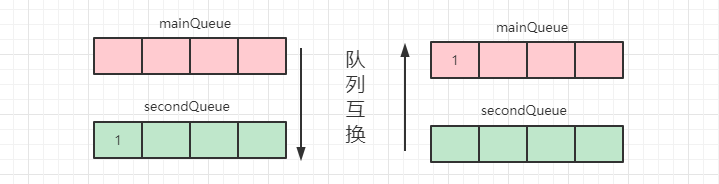1. 继续放入元素 2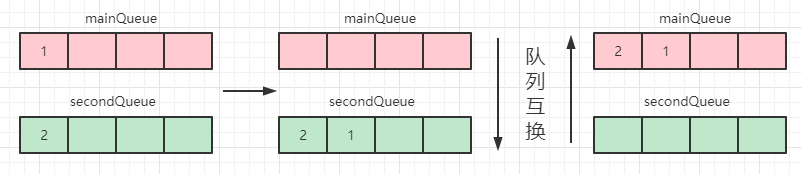1. 继续放入元素 3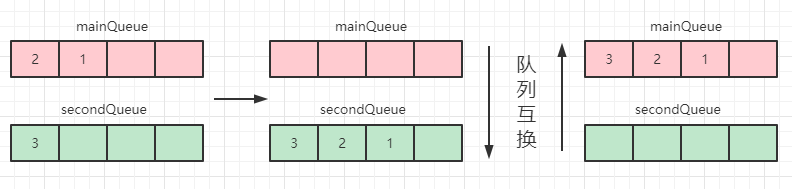package com.lonely.wolf.note.stack;

import java.util.Queue;

public class MyStackByTwoQueue {
public static void main(String[] args) {
MyStackByTwoQueue queue = new MyStackByTwoQueue();
queue.push(1);
queue.push(2);
System.out.println(queue.pop());
}

private Queue<Integer> mainQueue = new LinkedList<>();
private Queue<Integer> secondQueue = new LinkedList<>();

public void push(int e){
if (!mainQueue.isEmpty()){
}
//交换连个 queue，此时新加入的元素 e 即为 mainQueue 的头部元素
Queue temp = mainQueue;
mainQueue = secondQueue;
secondQueue = temp;
}

public int top(){
return mainQueue.peek();
}

public int pop(){
return mainQueue.poll();
}

public boolean empty() {
return mainQueue.isEmpty();
}
}


# 栈的经典应用场景

## 浏览器前进后退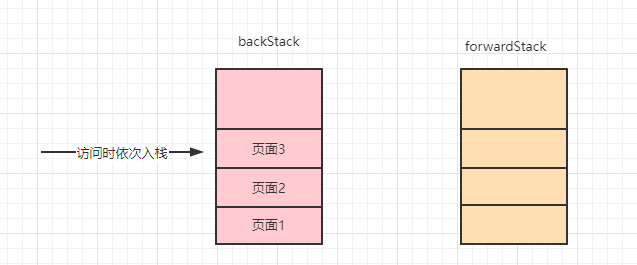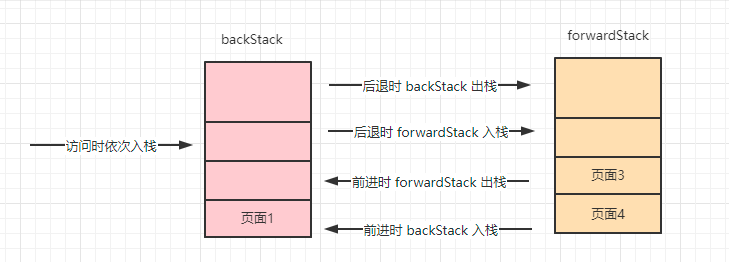## 括号配对

### leetcode 20 题

leetcode 中的第 20 题就是一道括号配对的题，题目是这样的：

public static boolean isValid(String s){
if (null == s || s.length() == 0){
return false;
}

Stack<Character> stack = new Stack<>();
Map<Character,Character> map = new HashMap<>();
map.put(')','(');
map.put(']','[');
map.put('}','{');
for (int i=0;i<s.length();i++){
char c = s.charAt(i);
if (c == '(' || c == '[' || c == '{'){
stack.push(c);//左括号入栈
}else{
if (stack.isEmpty() || map.get(c) != stack.peek()){
return false;
}
stack.pop();//配对成功则出栈
}
}
return stack.isEmpty();
}


## 表达式求值

1. 遍历表达式，当遇到操作数，将其压入操作数栈。
2. 遇到运算符时，如果运算符栈为空，则直接将其压入运算符栈。
3. 如果运算符栈不为空，那就与运算符栈顶元素进行比较：如果当前运算符优先级比栈顶运算符高，则继续将其压入运算符栈，如果当前运算符优先级比栈顶运算符低或者相等，则从操作数符栈顶取两个元素，从栈顶取出运算符进行运算，并将运算结果压入操作数栈。
4. 继续将当前运算符与运算符栈顶元素比较。
5. 继续按照以上步骤进行遍历，当遍历结束之后，则将当前两个栈内元素取出来进行运算即可得到最终结果。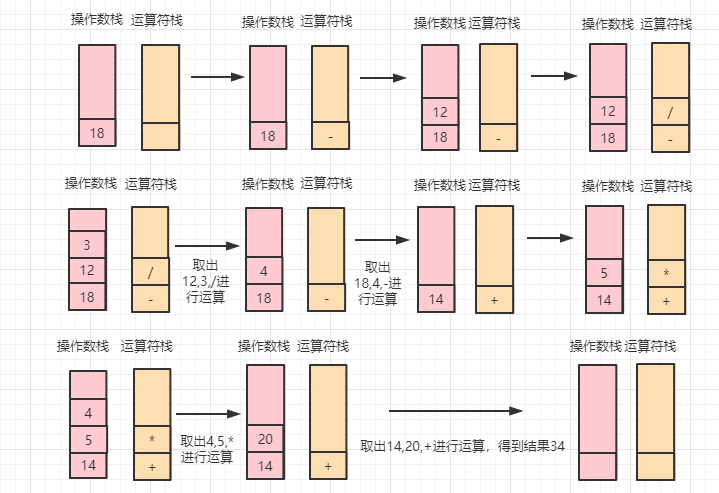### leetcode 227 题

1. 表达式中有空格，我们需要将空格处理掉
2. 操作数可能有多位，也就是说我们需要将操作数先计算出来。

#### 使用两个栈求解

public static int calculateByTwoStack(String s){
if (null == s || s.length() == 0){
return 0;
}
Stack<Integer> numStack = new Stack<>();//操作数栈
Stack<Character> operatorStack = new Stack<>();//运算符栈

int num = 0;
for (int i = 0;i<s.length();i++){
char c = s.charAt(i);
if (Character.isDigit(c)){//数字
num = num * 10 + (c - '0');
if (i == s.length() - 1 || s.charAt(i+1) == ' '){//如果是最后一位或者下一位是空格，需要将数字入栈
numStack.push(num);
num = 0;
}
continue;
}
if (c == '+' || c == '-' || c == '*' || c == '/'){
if (s.charAt(i-1) != ' '){//如果前一位不是空格，那需要将整数入栈
numStack.push(num);
num = 0;
}
if (c == '*' || c == '/'){//如果是乘除法，那么需要将当前运算法栈内的乘除法先计算出来
while (!operatorStack.isEmpty() && (operatorStack.peek() == '*' || operatorStack.peek() == '/')){
numStack.push(sum(numStack,operatorStack.pop()));//将计算出的结果再次入栈
}
} else {//如果是加减法，优先级已经是最低，那么当前运算符栈内所有数据都需要被计算掉
while (!operatorStack.isEmpty()){
numStack.push(sum(numStack,operatorStack.pop()));
}
}
operatorStack.push(c);
}
}
//最后开始遍历：两个操作数，一个运算符进行计算
while (!numStack.isEmpty() && !operatorStack.isEmpty()){
numStack.push(sum(numStack,operatorStack.pop()));//计算结果再次入栈
}
return numStack.pop();//最后一定剩余一个结果入栈了
}

private static int sum(Stack<Integer> numStack,char operator){
int num1 = numStack.pop();
int num2 = numStack.pop();
int result = 0;
switch (operator){
case '+':
result = num2 + num1;
break;
case '-':
result = num2 - num1;
break;
case '*':
result = num2 * num1;
break;
case '/':
result = num2 / num1;
break;
default:
}
return result;
}


#### 使用一个栈求解

• 加号：将数字直接压入栈内。
• 减号：将对应的负数压入栈内。
• 乘/除号：计算数字与栈顶元素，并将栈顶元素替换为计算结果。

public static int calculateOneStack(String s){
if (null == s || s.length() == 0){
return 0;
}
Stack<Integer> stack = new Stack<>();
char preOperator = '+';//默认前一个操作符是加号
int num = 0;
for (int i = 0;i<s.length();i++){
char c = s.charAt(i);
if (Character.isDigit(c)){
num = num * 10 + (c - '0');
}
if ((!Character.isDigit(c) && c != ' ') || i == s.length()-1){//判断数字处理是否已经结束，如果结束需要将数字入栈或者计算结果入栈
switch (preOperator){
case '+':
stack.push(num);//加法则直接将数字入栈
break;
case '-':
stack.push(-num);//减法则将负数入栈
break;
case '*':
stack.push(stack.pop() * num);//乘法则需要计算结果入栈
break;
case '/':
stack.push(stack.pop() / num);//除法则需要计算结果入栈
break;
default:
}
preOperator = c;
num = 0;
}
}
int result = 0;
while (!stack.isEmpty()){//最后将栈内所有数据相加即可得到结果
result+=stack.pop();
}
return result;
}


# 总结

posted @ 2022-01-10 12:52  双子孤狼  阅读(434)  评论(3编辑  收藏  举报